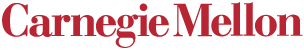10-602 CALD 15-802 Computer Science 36-712 Statistics 80-802 Philosophy Statistical Approaches to Learning and Discovery Course Web PageTime: Mondays and Wednesdays 1:00 pm - 2:20 pm

Location:  Wean Hall 4615A

Instructors:  Zoubin Ghahramani  (zoubin@cs.cmu.edu) and Teddy Seidenfeld (teddy@stat.cmu.edu)

Textbook:  Tanner's "Tools for Statistical  Inference", supplemented by readings.

Additional Reference Material:  G.Casella and R.Berger, "Statistical Inference." and  J.O.Berger, "Statistical Decision Theory" 1st ed. (only).  Both books are on "Reserve" in the E&S Library. The 2nd  edition of Berger is better, but was not in the library yesterday.  We'll try to get  the 2nd ed. on reserve once it shows up.

Written Requirements:  There will be approximately 5 homework assignments, a mid-term  examination, and a final examination.

## Tentative Topic List and Schedule

Here is a schedule of class meetings and a preliminary choice of topics to be covered.
We emphasize that the selection of topics and their order of appearance is tentative.
 Week 1 (Jan 14 and 16) Introduction, information theory and statistics Supervised vs Unsupervised vs Reinforcement Learning Statistics and Information theory Maximum Likelihood  Bayesian learning Lecture Slides Week 2 (Jan 23) Some asymptotics of Bayesian inference Asymptotic certainty Asymptotic consensus Lecture Slides (corrected) Tanner Chapter 2 Week 3 (Jan 28 and 30) Normal and other approximations to Bayesian inference Symmetry and independence assumptions Data reduction Asymptotic Normality Tanner Chapter 2 Lecture Slides (Monday) Lecture Slides (Wednesday) Week 4 (Feb 4 and 6) Latent variable models Mixture of Gaussians (MoG) and k-means Factor Analysis (FA) and PCA Relation to Neural Networks Lecture Slides(revised) Homework 1 (revised, due Wed Feb 13) Text data for Beta-binomial problem Matlab minimize function Matlab digamma function Week 5 (Feb 11  and 13) The EM algorithm General theory Missing data  Exponential Family Bayesian and non-Bayesian form Applications to MoG and FA Lecture Slides (Mon) Extended Remarks on Improper Priors Slide on KL Inequality Lecture Slides (Wed) Tanner Chapter 4 Week 6 (Feb 18 and 20) MCMC methods Simple Monte Carlo Rejection Sampling Importance Sampling Gibbs Sampling  Relation between Gibbs, MCMC and EM Lecture Slides (Mon) Gibbs sampling demo (needs plot_gaussian) Tanner Chapter 6 and additional readings Lecture Slides (Wed) Radford Neal's Technical Report Week 7 (Feb 25 and 27) MCMC methods EM as MM, a counterexample Metropolis Hybrid Monte Carlo and other topics in sampling EM as MM with an EM counterexample (Mon / Teddy) metropolis demos: one and two hybrid Monte Carlo demo Week 8 (March 4 and 6) Variational Methods and Probabilistic Graphical Models Variational Methods Conditional Independence Bayesian Networks (directed graphical models) d-Separation Lecture Slides (Mon) Jordan et al Variational Tutorial Homework 2 (part 1) Homework 2 (part 2) Data for Hw 2: images.jpg Data generating code: genimages.m Week 9 (March 11 and 13) Probabilistic Graphical Models and Causal Inference Markov Networks (undirected graphical models) Hammersley-Clifford Theorem Belief Propagation  Causal Inference Lecture Slides (Mon) Scheines, R. An Introduction to Causal Inference. Spirtes, P., Richardson, T., Meek, C., Scheines, R., and Glymour, C.  (1997). Using Path Diagrams as a Structural Equation Modelling Tool (Sociological Methods and Research) Scheines, R., Spirtes, P., Glymour, C., Meek, C., and Richardson, T.  (forthcoming). The TETRAD Project: Constraint Based Aids to Causal Model Specification (Multivariate Behavioral Research) Tetrad Software Project Homepage Week 10 /11 (March 18, 20, 25, 27) Latent Variable Time Series Models Hidden Markov Models (HMMs) Forward-Backward and Viterbi Linear Dynamical Systems Kalman Filtering (KF) and Extended KF Particle Filters Hybrid and Nonlinear Time Series Models Dynamic Bayesian Networks Paper on Learning Dynamic Bayesian Networks[pdf] [ps] Lecture Slides (Wed 20, Mon 25) . CMU SPRING BREAK . . Week 12 (April 8 and 10) Sample Reuse Techniques Jackknife Bootstrap Lecture Slides (Sample Reuse Techniques) Homework 3 (part 1) geyser.txt Week 13/14 (April 15, 17, 22) Reinforcement Learning and Sequential Decisions Decisions Active Learning Experiment Design Reinforcement Learning Markov Decision Problems The Bellman Equation Temporal Difference and Q-learning Relation to Optimal Control and Influence Diagrams Lecture Slides (Sequential Decision Making) Lecture Slides (Reinforcement Learning) Sutton and Barto Textboook Kaelbling, Littman and Moore Review Paper Week 14/15 (April 24, 29, May 1) Model Selection Bayes Factors Multiple Testing BIC/MDL Laplace Approximation and Variational Methods Cross Validation Lecture Slides (Bayesian Model Selection) Lecture Slides (Cross Validation)

Other topics we would have liked to cover:

Boosting
Iterative Scaling
AIC

Exact Sampling Methods

Hierarchical and Nonlinear Latent-Variable Models:

• Independent Components Analysis (ICA)
• Sigmoid Belief Networks
• Boltzmann Machines
Nonparametric Inference
• Kernel Methods
• Density estimation, classification and regression
• Gaussian processes as infinite neural networks, Bayesian kernels machines and priors over functions
• Dirichlet Processes and infinite mixture models
Loopy Belief Propagation, Bethe Approximations, and Kikuchi Approximations

Expectation Propagation